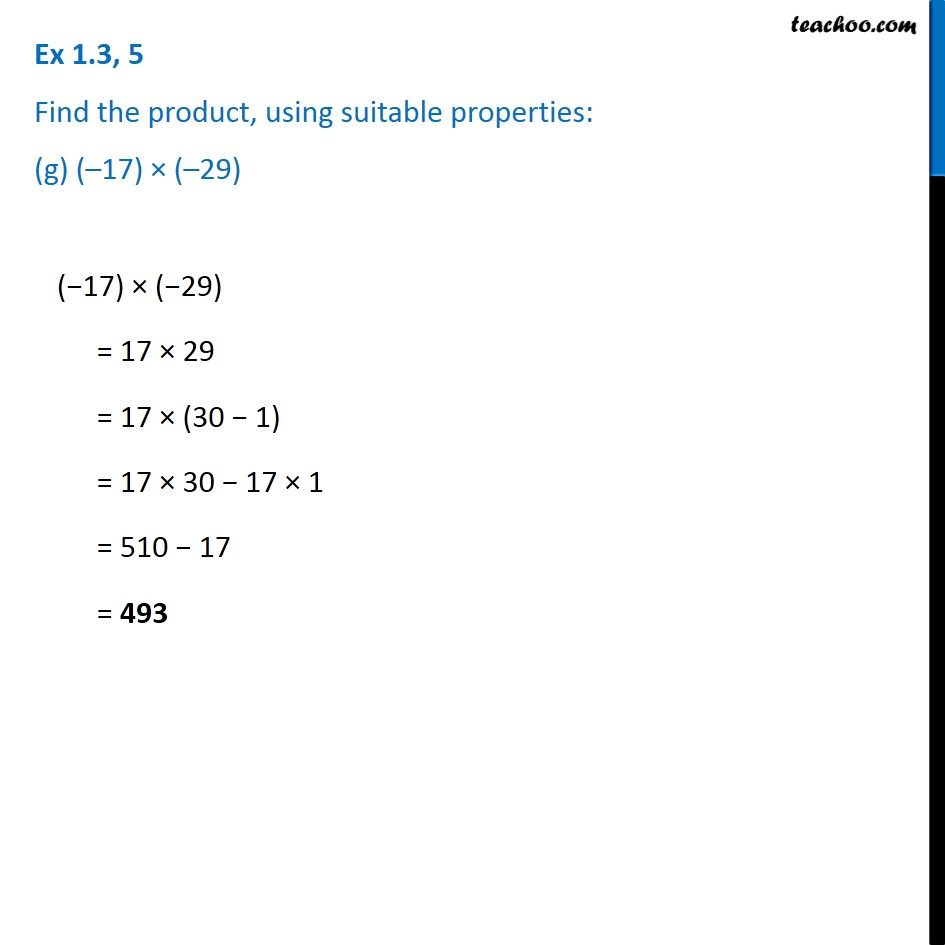1. Chapter 1 Class 7 Integers
2. Serial order wise
3. Ex 1.3

Transcript

Ex 1.3, 5 Find the product, using suitable properties: (g) (–17) × (–29) (−17) × (−29) = 17 × 29 = 17 × (30 − 1) = 17 × 30 − 17 × 1 = 510 − 17 = 493